# Maximum range on ground for an elevated cannon

• brotherbobby

#### brotherbobby

Homework Statement
A cannon sits atop on a plane at a height ##h## above the ground and fires a shell with an initial velocity ##v_0##. At what angle ##\theta_0## must it fire the shell so as to attain maximum range ##R_{\text{max}}## along the ground?

Ans : ##\boxed{\boldsymbol{\theta_0 = \sin^{-1} \frac{v_0}{\sqrt{2(v_0^2+gh)}}}}##
Relevant Equations
(1) Equation of motion of the shell in the vertical direction : ##y = h+ v_0 \sin\theta_0 t-\frac{1}{2} gt^2##.
(2) Equation of motion in the horizontal direction : ##x = v \cos \theta_0 t## for any time ##t##.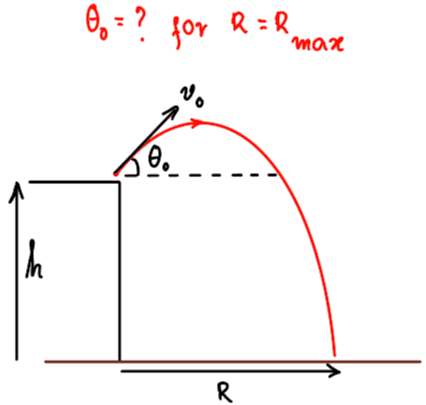I sketch a diagram for the problem and show it to the right.

My approach is going to be along the following lines : (1) Use the equation of motion in the ##x## direction to express the total time of flight ##T## as a function of the range and initial angle ##T = T(R, \theta_0)##. (2) Plug this value of time into the equation of motion in the ##y## direction to express the height ##h## as a function of range, gravity and initial angle ##h = h(R,g, \theta_0)##. (3) Express the range ##R## as a function of height, initial angle, gravity and initial velocity : ##R = R(h, \theta_0, v_0, g)##. (4) Differentiate the equation of ##R## with respect to ##\theta_0## and set it equal to zero and solve the resulting equation to express the initial angle as a function of height and initial velocity : ##\boxed {\theta_0 = \theta_0(v_0, h, g)}##. This should be the answer to the problem.

My attempt :

Range ##R = v_0 \cos\theta_0 T## where ##T## is the time period of flight. Hence ##T = \frac{R}{v_0 \cos\theta_0}##. Again, the height ##-h = v_0\sin\theta_0 T - \frac{1}{2}gT^2 \Rightarrow -h = R\tan\theta_0 - \left( \frac{gR^2}{2v_0^2}\right) \frac{1}{\cos\theta_0^2}##.
Simplifying and rearranging, this leads to a quadratic equation in ##R## : $$R^2 - \frac{v_0^2 \sin 2\theta_0}{g}R-\frac{v_0^2 h}{g}(1+\cos 2\theta_0)=0$$ Using the quadratic formula, we solve for ##R = \frac{v_0^2 \sin 2\theta_0}{2g}+\frac{1}{2g}\sqrt{\frac{v_0^4}{2}(1-\cos 4\theta_0)+4v_0^2 gh(1+\cos 2\theta_0)}##. Differentiating this with respect to ##\theta_0##, we obtain $$\frac{dR}{d\theta_0} = \frac{v_0^2 \cos 2\theta_0}{g}+ \frac{1}{2g\sqrt{\mathscr{E}}}(2v_0^4 \sin 4\theta_0-8v_0^2 gh \sin 2\theta_0)$$ where ##\mathscr E## stands for the expression inside the square root sign in the equation above. Setting this derivative to 0 for maximum range ##R_{\text{max}}## and writing the expression for ##\mathscr {E}##, we get the following equation: $$\cos 2\theta_0+\frac{v_0^2 \sin 4\theta_0-4gh \sin 2\theta_0}{\sqrt{\frac{v_0^2}{4}(1-\cos 4\theta_0)+4v_0^2gh(1+\cos 2\theta_0)}}=0\;\; \left(\frac{v_0^2}{g}\neq 0 \right)$$

The problem is, upon squaring and simplifying the above equation in order to get rid of the square root, this leads to a near impossible equation to solve in trigonometry after several lines of slightly tedious algebra: $$\frac{3}{8} \cos 8\theta_0-3v_0^2 gh \cos 6\theta_0 - (8g^2h^2-2v_0^2gh)\cos 4\theta_0 - 3v_0^2gh\cos 2\theta_0 - \left(\frac{3}{8} v_0^4 - 2v_0^2gh-8g^2h^2 \right) = 0$$

I am supposed to solve this equation in order to express ##\theta_0 = \theta_0(h, g, v_0)##, but I wonder if the above equation be solved.

A hint or help would be welcome.

For instance, do we have softwares (online or otherwise) that can solve trigonometric equations?

Last edited:

Rather than wade through your algebra to find an error (and there may be none), I found it much easier to go back to your quadratic in R and differentiate immediately. Setting dR/dθ to zero gives a linear equation in R which you can then substitute back into the quadratic.
Working in terms of 2θ is reasonable, to start with, but I would resist going to higher multiples.

Sorry for the delay, but I have good news. Please see through my calculation when you have the time, specially my finding of the maximum range ##R_{\text{max}}##.

We had the following quadratic equation for the range ##R## (see above) : $$R^2 - \frac{v_0^2 \sin 2\theta_0}{g}R-\frac{v_0^2 h}{g}(1+\cos 2\theta_0)=0$$

I type the remaining using a combination of MSWord and MathType for faster typing. I hope am not violating anything.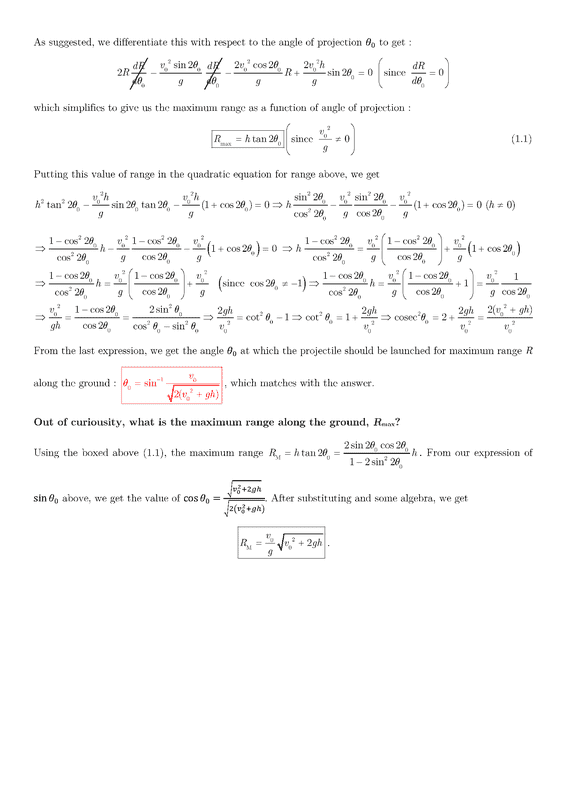Is my working correct above? Sorry for the poor size of the image.

•Delta2
Well done.
I found a slightly shorter route..
Assuming all trig functions refer to 2θ:
##\tan^2=\frac{v^2}{gh}(1+\cos+\sin \tan)##
##\sin^2=\frac{v^2}{gh}(\cos+\cos^2+\sin^2)\cos##
##1-\cos^2=\frac{v^2}{gh}(1+\cos)\cos##
giving us a quadratic in cos 2θ

Thank you. However, my problems don't end there. The author whose book I am following has given a shorter way to do the problem which, for all its elegance, leaves me wondering and confused on several points. I will copy and paste the author's solution and marks appropriate places blue asterisks (*) which I get and red question marks (?) for places where am confused. I will go on to explain what my confusions are.

Author's question and solution : (sorry for the cloudy font and text)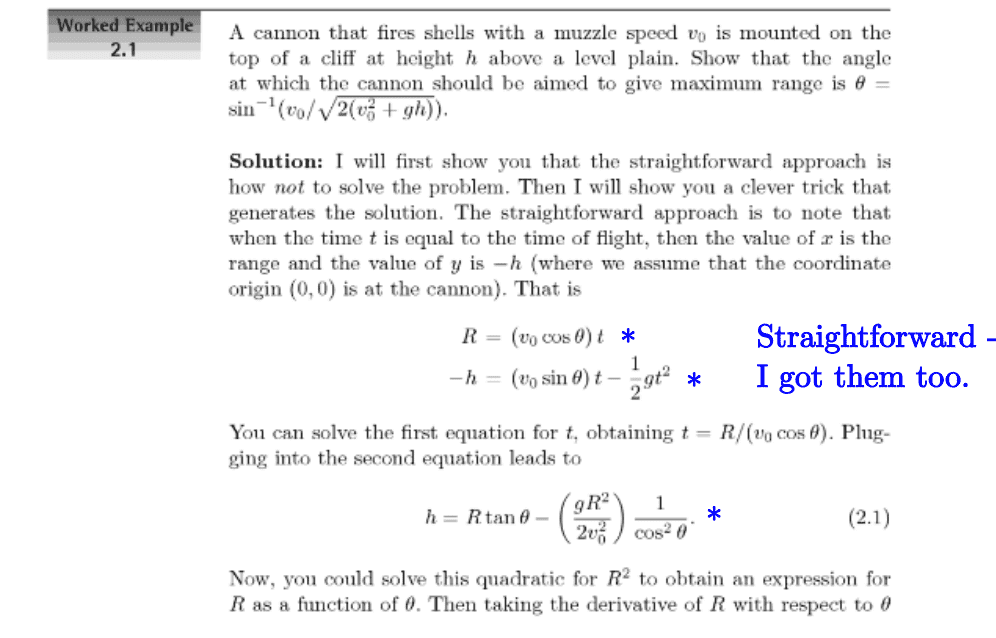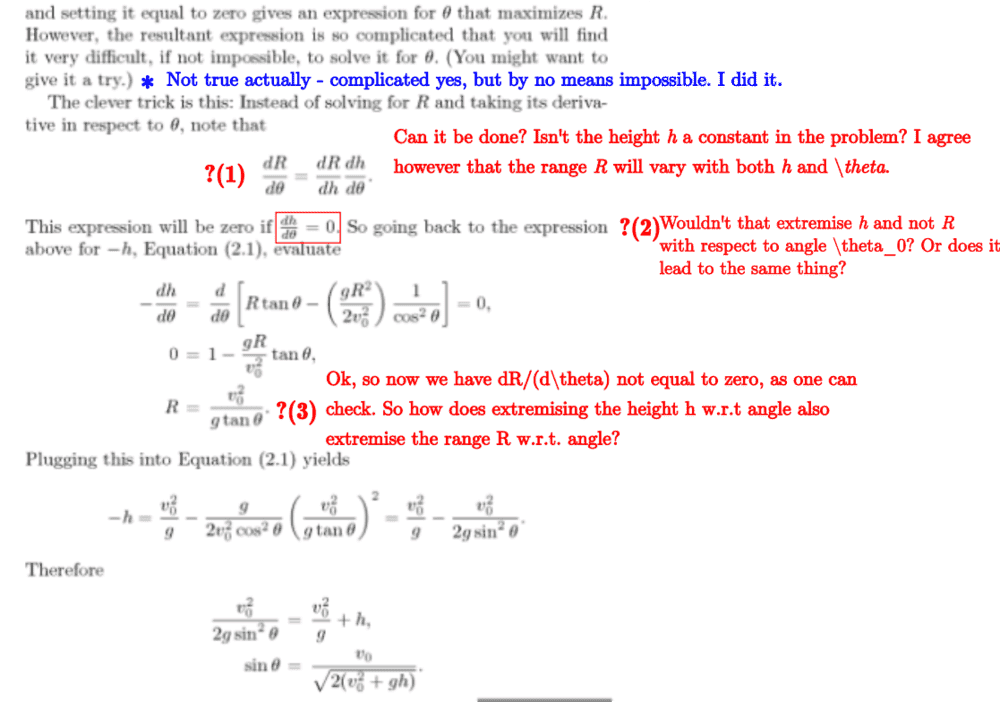•Delta2
The "trick" I'd use is to set ##u = \tan \theta##: $$\frac{gR^2}{2v^2}(1 + u^2) - Ru = h$$ Then implicitly differentiate wrt ##u##, setting ##\frac{dR}{du} = 0##, giving: $$R = \frac{v^2}{gu} = \frac{v^2}{g\tan \theta}$$ Then you plug that into the first equation to give: $$\tan^2 \theta = u^2 = \frac{1}{1 + 2gh/v^2}$$ Then use a bit of trig to get that in terms of ##\sin^2 \theta##.

Last edited:
•haruspex
I'm with you, the reasoning given is quite invalid.
But the algebra worked for a different reason. In differentiating (2.1), since h is constant, dh/dθ=0, so choosing to set it zero is fine. On the RHS, the full form of the derivative would include dR/dθ terms, but since we want the extreme of R wrt θ these go to zero. Consequently the equation obtained is valid. Indeed, this turns into exactly the method I used.

An infrequently used, but definitely useful and always applicable, equation for the range in terms of the initial and final velocities of the projectile is, $$R=\frac{|\vec v_0\times \vec v_f|}{g}.$$Clearly, the range is maximized when the numerator is the product of the initial and final speeds. The angle of projection at maximum range is easily found using geometry and the basic definitions of trigonometric functions. If interested, see this insight for details including a bag of geometric tricks applied to projectile motion.

Last edited:
The 'hard yards' for this solution are shown in the following very excellent set of notes on the kinematics of projectiles on an inclined plane:

https://cnx.org/contents/UYPplaH7@29.32:--TzKjCB@8/Projectile-motion-on-an-incline

The following expression for range down an inclined plane with slope angle ##\alpha## is given:

$$R=\frac{u^2}{g\cos^2\alpha}\left(sin(2\theta+\alpha)+sin\alpha\right)$$

Maximization is easily obtained by setting ##2\theta+\alpha=\frac{\pi}{2}## whence ##\theta_{max}=\frac{\pi}{4}-\frac{\alpha}{2}.##Then $$R_{max}=\frac{u^2}{g\cos^2\alpha}\left(1+\sin\alpha\right)=\frac{u^2}{g(1-\sin\alpha)}.$$ Substitute $$R_{max}=\frac{h}{\sin\alpha}$$ leading to $$\sin\alpha=\frac{hg}{hg+u^2}.$$ ##\tan\theta_{max}## and hence ##\sin\theta_{max}## can be obtained using the useful identity: $$\tan\left(\frac{\pi}{4}-\frac{\alpha}{2}\right)=\frac{1-\sin\alpha}{\cos\alpha}$$

Last edited:
•PeroK
That's a different problem: that's an incline, not a cliff.
Just draw in the 'incline' from cliff top to point of impact !

•PeroK
Just draw in the 'incline' from cliff top to point of impact !
Is that before or after you've calculated the range off the cliff?

Before - in the workings I show how to calculate ##R_{max}##.

What confuses is that my ##R{max}## is the hypotenuse of the triangle. But maximising this will also maximise the horizontal range subject to the constraint of a constant height.

What confuses is that my ##R{max}## is the hypotenuse of the triangle. But maximising this will also maximise the horizontal range subject to the constraint of a constant height.
Let's see the calculation. You can't leave an answer in terms of an unknown angle ##\alpha##.

The correct answer is: $$R = \frac v g \sqrt{v^2 + 2gh}$$
Where ##R## is the maximum horizontal range. I don't see how you are getting that.

Last edited:
Let's see the calculation. You can't leave an answer in terms of an unknown angle ##\alpha##.
Certainly:

$$\tan\left(\frac{\pi}{4}-\frac{\alpha}{2}\right)=\frac{1-\sin\alpha}{\cos\alpha}$$
$$\sin\left(\frac{\pi}{4}-\frac{\alpha}{2}\right)=\frac{1-\sin\alpha}{\sqrt{2-2\sin\alpha}}=\sqrt{\frac{1-\sin\alpha}{2}}=\sqrt{\frac{u^2}{2(hg+u^2)}}$$ $$=\frac{u}{\sqrt{2(hg+u^2)}}$$

The correct answer is: $$R = \frac v g \sqrt{v^2 + gh}$$
Where ##R## is the maximum horizontal range. I don't see how you are getting that.
My ##R_{max}=\frac{h}{\sin\alpha}.## So the corresponding horizontal ##R_{max}=h\cot\alpha## $$h\cot\alpha=\frac{h u\sqrt{u^2+2hg}}{hg}=\frac{u\sqrt{u^2+2hg}}{g}$$

I concur that it does. You can see that from the expression for the range in post #8. Maximum range is obtained when the final and initial velocities are perpendicular.$$R_{\text{max}}=\frac{v_0v_f}{g}$$ From energy conservation ## v_f=\sqrt{v_{0}^2+ +2gh}##. Then $$R_{\text{max}}=\frac{v_0\sqrt{v_{0}^2 +2gh}}{g}.$$

•neilparker62
Yes, thanks.

•kuruman
What confuses is that my ##R{max}## is the hypotenuse of the triangle. But maximising this will also maximise the horizontal range subject to the constraint of a constant height.
Okay, I see this now. That's certainly another way to get the answer.

•neilparker62
To complete the picture, when the range is maximum, the initial and final velocity vectors form a right triangle as shown below.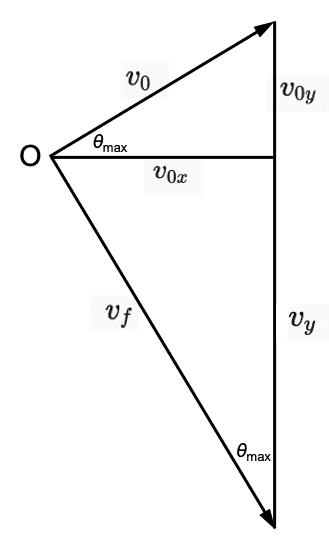From the velocity triangle, $$\tan(\theta_{\text{max}})=\frac{v_0}{v_f}=\frac{v_0}{\sqrt{v_0^2+2gh}}.$$ Applying the trig identity for the sine in terms of the tangent verifies the answer posted in #1. It is interesting to note that the product $$R_{\text{max}}\tan(\theta_{\text{max}}) =\frac{v_0^2}{g}$$ is independent of the height of the cliff ##h##.

•neilparker62 and PeroK
The 'hard yards' for this solution are shown in the following very excellent set of notes on the kinematics of projectiles on an inclined plane:

https://cnx.org/contents/UYPplaH7@29.32:--TzKjCB@8/Projectile-motion-on-an-incline
Those notes make a terrible meal of things. For a projectile fired at initial speed ##v## at an angle ##\theta## to the horizontal, along an incline of angle ##\alpha## (which may be +ve or -ve), where the point of impact is ##(x, y)## and the distance along the incline is ##d##, with ##d^2 = x^2 + y^2##:
$$y = x\tan \theta - \frac{gx^2}{2v^2}\sec^2 \theta \ \implies \ \tan \alpha = \frac y x = \tan \theta - \frac{gx}{2v^2}\sec^2 \theta$$ Which gives:
$$x = \frac{2v^2}{g}\cos^2 \theta(\tan \theta - \tan \alpha)$$ $$y = x\tan \alpha$$ $$d = \frac{2v^2}{g}\cos^2 \theta(\tan \theta - \tan \alpha)\sec \alpha$$ And, the time of flight is:
$$t = \frac{x}{v\cos \theta} = \frac{2v}{g}\cos \theta(\tan \theta - \tan \alpha)$$
To maximise ##d## we need to maximise $$\sin \theta \cos \theta - \tan \alpha \cos^2 \theta$$ which gives:
$$\tan 2 \theta = - \cot \alpha$$ which is equivalent to $$\tan \theta = \tan \alpha + \sec \alpha$$
That gives us the maximum range in terms of ##x## or ##d##:
$$x_{max} = \frac{v^2}{g}(\sec \alpha - \tan \alpha) = \frac{v^2}{g}\big ( \frac{\cos \alpha}{1 + \sin \alpha} \big )$$
$$d_{max} = \frac{v^2}{g}\big ( \frac{1}{1 + \sin \alpha} \big )$$